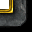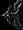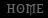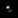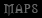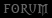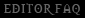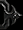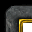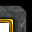# Mathematics - Functions

Tutorial By martix

Foreword
This tutorial is about all the mathematic functions and possibilities that exist withing the World Editor. Its aimed at begginers as well as advanced users. And its also an effort to prove that what you learn(ed) in math classes in school can be useful.

I. Introduction
II. Basic Definitions and General Stuff
III. Algebraic Funtions
IV. Geometric/Trigonometric Functions

I. Introduction
This is a comprehensive tutorial that (if you are willing to read it through) will teach you about all the possibilities for working with numbers when using the World Editor. I guess the algebra(on the level WE offers it) will seem easy enough, but trigonometry may be a diffrent thing(I wasn't good at it either). One thing I'm saying from the beggining - I'm not going to (re)teach all the maths - thats something you learn in school, not in a WE tutorial! With that said lets start with the basics you need to know.

II. Basic Definitions and General Stuff

1. Math functions are used in triggers to calculate diffrent numbers and if used to the full potential can be a priceless asset in creating a great trigger enchanced spell or some other original and/or fancy feature in your map, that would make it outstanding. I guess anyone who has brains enough to understand this tutorial would also have enough sense of logic to be able to understand triggers quite well.

2. Variables - I'm gonna make a quick explanation, there are 2 types of numeric variables in the World Editor:

a) An Integer variable - this variable can take ONLY whole(positive or negative) numbers(like counting units and other things that can never have a decimal). Thats why there are less math functions with it and also no trigonometric functions at all. An integer can assume any value between -2147483648 to 2147483647.(Thanks wonderpriest).

b) A Real variable - this variable can take any real value, be it positive, negative, whole or decimal. The accuracy is up to the second digit(one hundredth). But since this is math and everything is equal to something else, in some cases there is a simple way to achieve more accuracy(if needed), I'll talk about that later. Its also a reqirement for anything involving geometry in the World Editor.
Note: Here's something odd I am not sure of - in the editor, the accuracy goes to the 2nd digit after the decimal point, in the game it goes 3rd, and I'm not sure if this 3rd digit can actually be used.

3. Some Specifics and notes:
a) Watch the titles - Each window that reqires some sort of number - pay heed to the title of that window saying what type of number it requires (integer or real), so you don't have to wonder why you can't find the function you need. There are a lot of common functions, but each type has functions that are specific to it. Example - any type of counting function is restricted to integers, and most of the unit properties(life, movement speed, etc.) are Real-only accesible. I will be noting that troughout this tutorial, functions that are not marked as "Real-only" are accesible to every type.

b) Conversions - But there is a way around that - with the conversions, they can convert one number type to another. A word of warning though - not all conversions are reasonable, despite still being possible. One particular combination you should ALWAYS avoid is Integers+Trigonometry!
Note:When converting reals to integers the real is not rounded. Like 9.8 &#8776; 10 - how normal rounding would work. Instead the decimal part is quite simply cut off! In this case 9.8 becomes 9. Remember this! (Credit to Luth for this specification)

c) Percents - a percent is also a predefined number. 100%=1 - you gotta remember that simple fact. You can get the percent of a number simply by multiplying it with some other number. For example - 69% of 246= 0.69*246. Its as simple as that.

d) When you encounter some difficulty of mathematical nature I strongly suggest you leave the computer be, take a sheet of paper and a pencil approach the problem not as a part of your trigger(or your map), but as a maths task you were given to solve. Believe me it helps. It is when you solve it that you can start thinking of a way to implement it in your map.

III. Algebraic Functions

1. Arithmetic
This is the first and most simple math operation in the editor. You got 2 number, no matter how you got them(by direct input or as a result of another function) and one of 4 operations you all know - addition, sustraction, multiplication and division. Nothing more to say about that really.
Example - 2+2=4 (or is it :) ) Well, as simple as that...

2. Math - Random Number
This fuction returns a random number in the numerical range you specify of the type that is required. A random real number will almost always return a number with a decimal part(there is only one hundredth - 1% chance it returs a non-decimal) :) It is the only function that returns a random number in a user-defined range.

3. Math - Random Angle *(Real-ONLY)
This one also returns a number, but in a predefined range - 0 to 360, the value of a full circle. And btw, in this case - 0=360

4. Math - Random Percentage *(Real-ONLY)
Returns a REAL value between 0 and 100 to be used as a percentage(nothing more to say I guess).

Note: I have one very imporant thing to add about randomness though - I've seen a lot of threads and questions asked about why I got the same hero N times in a row and why did that guy score 5 consecutive criticals(and probably killed you :) )
There are ways to generate absolutely random numbers, but computer algorithms cannot be used for that, since, by definition, no deterministic mathematical algorithm can generate a random result.
Do remember this whenever a question of the above type comes to your mind!

5. Math - Min
This is used to compare 2 numbers and give the lesser one. The position doesn't matter. It always involves at least variable(or you banged your head and don't know if 1 is bigger than 2) :)
Example: Min(5,2) would return 2 as it is lesser than 5.

6. Math - Max
The opposite of the above one, only returns the greater value of the values you input. Again, it doesn't matter if its value 1 or 2.
Example: Max(6,12) would return 12 as it is bigger than 6.
These two for example can be used ot avoid certain conditionals and save you a bit of time.

7. Math - Abs
It returns the absolute value of the given number.
3=3, 4.25=4.25, -7=7, -43.5=43.5
To illustrate imagine an axis with all numbers placed on it - this returns the distance to that number from the 0, no matter in which direction you go(positive or negative). (Wonder - did you imagine anything, and if you did, was it remotely similar to mine?) :D

8. Math - Sign
You can think of this as the opposite of the above function.
It gives you(returns) the sign of the number you input. But since it has to spit out a number, it gives you 1 or -1.
12=1 or -32=-1

9. Math - Modulo
Calculates the remainder of a devision.(That would be enough for me, but who knows what people are out there).
It gives you what is left behind when the division reaches the decimal point. Divisions that return a whole number do not have a remainder.
This is a very useful, yet underused function. It can be used for ex. when going from one numeric system to another(decimal->hex->octal).

10. Math - Power *(Real-ONLY)
You give a number and a power and it gives you back that number raised to that power. Any number and power can be used, the power value is also real, which means it can have a decimal part.

11. Math - Square Root *(Real-ONLY)
It calculates the square root of a number and only the square one(maybe blizzard doesn't like the other shapes :) )
sqrt(X^2)=X
As suggested you can use this to calculate whichever root you want. This can be achieved very simply by making use you certain exponential identities. Just look at the link(s) posted at the end.

These are pretty much all the algebraic functions, now comes Trigonometry(the advanced stuff).
Some notes - using the arithmetic function you can actually get past the 2 digit decimal limitation when using predefined numbers(ones you typed yourself, not changeable). For example - 943.89/100=9.4389 - thats how you can simulate a more accurate number. :)

IV. Trigonometry/Geometry
*(Real-ONLY)

Every function here is a definate REAL-only. Most of the trigonometric functions always return a decimal value. And for example sine and cosine ones are always between 1 and -1. So DO NOT EVER convert these to integers! You'll end up with weird results AT BEST.

1. A random definition from the web:

Trigonometry - Study of triangles, the measurements of their parts and of angle functions and relations.

2. Presets: You can set a real variable any value you want in the variables window. There are also presets, 2 of which are worth explaining - pi and e
a) pi&#8776;3.1415926536 (Believe me or not, but I didn't look it up anywhere, I just remember it to the 10th digit :) The most accurate(if I remember correctly) is 22/7
b) e &#8776; 2.71828 18284 59045 23536
This is also one of the most important mathematical constants, for more info visit wikipedia at http://en.wikipedia.org/wiki/E_%28mathematical_constant%29
There you will find a simple enough explanation(for me at least).

3. Conversions - Convert degrees to radians and vise versa. To understand these, you gotta understand both definitions.
Degrees - Any positive real number between 0 and 360
Radian - One radian is the angle of an arc created by wrapping the radius of a circle around its circumference. The radius 'r' fits around the circumference of a circle exactly 2p times. That is why the circumference of a circle is given by circumference = 2pi*r. So there are 2pi radians in a complete circle, and pi radians in a half circle. Degrees=Radians*180/pi

4. Math - Random Angle
Same as above, wanted to put all random things in one place.

5. Math - Distance between points
This function gives you the distance between 2 points in pixels, warcraft's default distance measurement unit(move speed is also measured in pixels per second a unit can travel).

6. Math - Angle between points
This may seem a little odd the first time(maybe impossible too :) ) But say you got point A and B. If point A is the center of a circle, the distance between the two points is the radius of that circle and point B is on the circumference of the circle, then the angle is equal to the position of B on that circle in reference to WC's coordinate system where 0 degrees is east, 90 is north, 180 is west.
So if point B is directly vertically positioned above A, then this function would return 90 degrees.

7. Math - Sine
This calculates the sine of a given angle. The sine of an angle in a right triangle equals the opposite side divided by the hypotenuse. See the attached picture.

8. Math - Cosine
This is the opposite of the above function, calculates the cosine of a given angle. In a right triangle the cosine of an angle equals the adjacent side divided by the hypotenuse. Again see the picture. These two functions are always in the range of -1 to 1, ALWAYS!
Oh and if you must know - sine^2+cos^2=1 always - this is the most basic trigonometric equation.

9. Math - Tangent
Calculates the tangent of a given angle. The tangent is the link between the above 2 functions and is equal to (sine of that angle)/(cosine of that angle). In a right triangle the tangent is the opposite side divided by the adjacent side.
Note: there is another function - the cotangent, which is the opposite(cosine/sine), but its not included since they are muttually interchangeable(1/tangent=cotangent).

These were the "straight" functions, now come their inverse counterparts, arc functions. They are usually denoted by a -1 superscript(like sin raised to power -1).

10. Math - Arcsine (or inverse sine)
This takes a sine value and converts it to its corresponding value in degrees. See Math - Sine for explanation of the function. Input range: -1 to 1, output range is -90 to 90 degrees,

11. Math - Arccosine (or inverse cosine)
Takes a cosine value and returns its value in degrees. Input range: -1 to 1, Output range - 0 to 180 degrees.

12. Math - Arctangent from angle (or inverse tangent)
Takes a tangent value and returns its value in degrees. Input range: -inf to inf, output range is -90 to 90 degrees.

13. Math - Arctangent from deltas (or inverse tangent)
This requires a diffrent input. It needs 2 deltas as input. A delta is essentialy one of the components(X or Y) of a vector. Input range: -inf to inf, output range is -180 to 180 degrees.
Er, how exacly to explain this simply...

Say you got one unit thats standing still and another unit thats running around it. The first unit is the beggining of the vector, the second is the end. If you put this function in the 1st units facing and supply the X and Y component of the resulting vector, this unit will always be facing the second one. Fair enough? (I'm a little rusty, so don't be mad at me).

Curious facts: I made the effort of searching for applications of these functions in Blizzards maps(campaign ones). I discovered that from the trigonometric ones only sine and cosine are used in the same trigger in several maps that involved caravans. It was just copied over and over for each map(even the name stayed the same). Actually I discovered that quite a few campaing triggers had their copies in a number of maps. :)

So, that would be all the mathematic functions in the editor and thus the end of my first tutorial. How you use all this is up to your imagination and knowledge. Post any comments and suggestions, there is always room for improvement.

As I said in the beggining, I wasn't planning on repeating your school curriculum(skipping that is/has been up to you alone), but it has been suggested that I include some formulas and stuff, so I'll just add a few links:
http://math2.org/ - Your general math tables. Here you can find everything from simple Polynomial Identities to Calculus.
http://www.lippsphysics.com/physics/formulas.html - Some physics and a funny comic strip.
http://www.lippsphysics.com/classes/syllabusdocs/equations.pdf - a PDF document containing anything math in nature you heart desires - syntesizing everything from nuclear physics and optics to trigonometry and calculus in one handy format.

Hope this has helped you :)